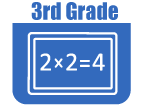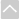OER Curriculum

These Open Educational Resources are comprehensive and coherent curricular materials that may be used to teach a course or grade level.

Engage NY
• Grade 3 Math Module 1: Properties of Multiplication & Division & Solving Problems with Units of 2-5
This 25-day module begins the year by building on students' fluency with addition and knowledge of arrays.
• Grade 3 Math Module 2: Place Value and Problem Solving with Units of Measure (EngageNY)
Module 2 uses place value to unify measurement, rounding skills, and the standard algorithms for addition and subtraction. The module begins with plenty of hands-on experience using a variety of tools to build practical measurement skills and conceptual understanding of metric and time units. Estimation naturally surfaces through application; this transitions students into rounding. In the modules final topics students round to assess whether or not their solutions to problems solved using the standard algorithms are reasonable.
• Grade 3 Math Module 3: Multiplication & Division w/ Units of 0, 1, 6-9, and Multiples of 10
This 25-day module builds directly on students work with multiplication and division in Module 1. Module 3 extends the study of factors from 2, 3, 4, 5, and 10 to include all units from 0 to 10, as well as multiples of 10 within 100. Similar to the organization of Module 1, the introduction of new factors in Module 3 spreads across topics. This allows students to build fluency with facts involving a particular unit before moving on. The factors are sequenced to facilitate systematic instruction with increasingly sophisticated strategies and patterns.
• Grade 3 Math Module 4: Multiplication and Area (EngageNY)
In this 20-day module students explore area as an attribute of two-dimensional figures and relate it to their prior understandings of multiplication. Students conceptualize area as the amount of two-dimensional surface that is contained within a plane figure. They come to understand that the space can be tiled with unit squares without gaps or overlaps. They make predictions and explore which rectangles cover the most area when the side lengths differ. Students progress from using square tile manipulatives to drawing their own area models and manipulate rectangular arrays to concretely demonstrate the arithmetic properties. The module culminates with students designing a simple floor plan that conforms to given area specifications.
• Grade 3 Math Module 5: Fractions as Numbers on the Number Line (EngageNY)
In this 35-day Grade 3 module, students extend and deepen second grade practice with "equal shares" to understanding fractions as equal partitions of a whole. Their knowledge becomes more formal as they work with area models and the number line.
• Grade 3 Math Module 6: Collecting and Displaying Data (EngageNY)
This 10-day module builds on Grade 2 concepts about data, graphing, and line plots. The two topics in this module focus on generating and analyzing categorical and measurement data. By the end of the module, students are working with a mixture of scaled picture graphs, bar graphs, and line plots to problem solve using both categorical and measurement data.
• Grade 3 Math Module 7: Geometry and Measurement Word Problems (EngageNY)
This 40-day final module of the year offers students intensive practice with word problems, as well as hands-on investigation experiences with geometry and perimeter. The module begins with solving one- and two-step word problems based on a variety of topics studied throughout the year, using all four operations. Next students explore geometry. Students tessellate to bridge geometry experience with the study of perimeter. Line plots, familiar from Module 6, help students draw conclusions about perimeter and area measurements. Students solve word problems involving area and perimeter using all four operations. The module concludes with a set of engaging lessons that briefly review the fundamental Grade 3 concepts of fractions, multiplication, and division.
•Georgia Standards of Excellence Curriculum Frameworks
• Grade 3 Unit 1: Number and Operations in Base Ten (Georgia Standards)
In this unit, students will investigate, understand, and use place value to manipulate numbers. Build on understanding of place value to round whole numbers. Continue to develop understanding of addition and subtraction and use strategies and properties to do so proficiently and fluently. Draw picture graphs with symbols that represent more than one object. Create bar graphs with intervals greater than one and use graphs and information from data to ask questions that require students to compare quantities and use mathematical concepts and skills.
• Grade 3 Unit 2: The Relationship Between Multiplication and Division (Georgia Standards)
In this unit, students will begin to understand the concepts of multiplication and division, learn the basic facts of multiplication and their related division facts, apply properties of operations (commutative, associative, and distributive) as strategies to multiply and divide, understand division as an unknown-factor problem. For example, find 32 8 by finding the number that makes 32 when multiplied by 8. Fluently multiply and divide within 100, using strategies such as the patterns and relationships between multiplication and division, understand multiplication and division as inverse operations, solve problems and explain their processes of solving division problems that can also be represented as unknown factor multiplication problems and represent and interpret data.
• Grade 3 Unit 3: Patterns in Addition and Multiplication (Georgia Standards)
In this unit, students will understand concepts of area and relate area to multiplication and addition. Find the area of a rectangle with whole- number side lengths by tiling it. Multiply side lengths to find areas of rectangles with whole-number side lengths in context of solving real world and mathematical problems. Construct and analyze area models with the same product. Describe and extend numeric patterns. Determine addition and multiplication patterns. Understand the commutative propertys relationship to area. Create arrays and area models to find different ways to decompose a product and use arrays and area models to develop understanding of the distributive property.
• Grade 3 Unit 4: Geometry (Georgia Standards)
In this unit students will further develop understandings of geometric figures by focusing on identification and descriptions of plane figures based on geometric properties. Identifies examples and non-examples of plane figures based on geometric properties. Identify differences among quadrilaterals. Understand that shapes in different categories may share attributes and those attributes can define a larger category (example: rhombuses, rectangles, and others have four sides and are all called quadrilaterals). Expand the ability to see geometry in the real world and can draw plane figure shapes based on attributes.
• Grade 3 Unit 5: Representing and Comparing Fractions (Georgia Standards)
In this unit, students will develop an understanding of fractions, beginning with unit fractions. View fractions in general as being built out of unit fractions, and they use fractions along with visual fraction models to represent parts of a whole. Understand that the size of a fractional part is relative to the size of the whole. For example, 1/2 of the paint in a small bucket could be less paint than 1/3 of the paint in a larger bucket, but 1/3 of a ribbon is longer than 1/5 of the same ribbon because when the ribbon is divided into 3 equal parts, the parts are longer than when the ribbon is divided into 5 equal parts. Students are able to use fractions to represent numbers equal to, less than, and greater than one.
• Grade 3 Unit 6: Measurement (Georgia Standards)
In this unit students will tell and write time to the nearest minute and measure time intervals in minutes. Solve elapsed time, including word problems, by using a number line diagram and reason about the units of mass and liquid volume.
•The Online Core Resource pages are a collaborative project between the Utah State Board of Education and the Utah Education Network. If you would like to recommend a high quality resource, contact Trish French (Elementary) or Lindsey Henderson (Secondary). If you find inaccuracies or broken links contact resources@uen.org.